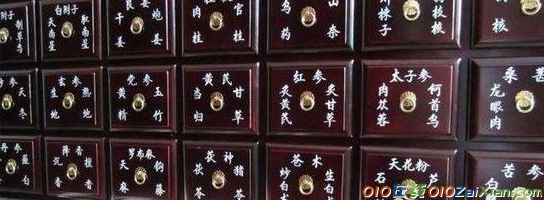## 药名谜语大全经典篇

药名谜语大全经典篇

日中煮茗(午时茶)/ 开绿灯(路路通)/ 千年裘(陈皮)/

读罢群书增见识(益智)/ 五月十五日(半夏)/ 酸甜苦辣咸(五味子)/

大路上摔筋斗(滑石)/ 离乡背井(生地)/ 仓库潮润(谷芽)/

岭石上的青苔(地骨皮)/ 人人都说家乡好(熟地)/ 台湾同胞盼统一(当扫)/

飞行员关机仓盖(防风)/ 三九时节(天冬)/ 哄堂大笑(合欢)/

偷梁换柱(木贼)/ 昭君出塞(王不留行)/ 九死一生(独活)/

故乡山水(熟地)/ 荒土(生地)/ 妇女节前一天(三七)/金链接铜链(黄连)/ 瀑布(泽泻)/ 戈壁林带(防风)/

出外之人思故乡(当归)/ 光荣标志(红花)/ 是草长年不发育(甘草)/

暑季自萎黄(夏枯草)/ 必欲先睹而后快(急性子 一见喜) 茅塞顿开(脑立清)/

留芳千古(安息香)/ 万物齐眠(全竭)/ 故乡(熟地)/相思病(没药)/

二十一(三七)/ 包龙图(首乌)/ 爸爸已到四十岁，养个儿子才八岁(五倍子)/胸怀大目标(远志)/ 事事如意(百合) 团聚——堂(合欢)/

沙漠造林(防风)/ 仙乐(神曲) / 请假期满(当归)/

自卫还击(防己)/ 决心扎根边疆(远志)/ 忠诚老实(厚朴)/

红光满面(丹皮)/ 一钱两用(二花)/ 陨石(天落星)/

中伏(半夏)/ 多读书、读好书(益智)/ 幸存一株英(独活)/

妇产房(生地)/ 焊接(续断)/ 老骥伏枥(远志)/

一令又一令(千张纸) 仙人之歌(神曲)/ 孤零的一生(独活)/

酣睡(安息香)/ 条条大道无阻挡(路路通)/ 读书好处多(益智)/

匿藏和尚(密佗僧)/ 自我戒备(防已)/ 家喻户晓(贯众)/

天女撒花(降香)/ 千里驹(马宝)/ 枯木逄春(苏木)/

低头思故乡(怀熟地)/ 不生第二胎(杜仲)/ 蜀币(川贝)/

一斗(亩合)/ 非同凡响(神曲)/ 初产绝育(杜仲)/

破屋更遭连夜夜雨(漏芦)/ 月经来潮(月月红)/ 牧童(牵牛子)/

四通八达(路路通)/ 寿比南山不老松(千年键)/ 飞骑穿杨(走马箭)/

盾(卫矛)/ 浪子回头(金不换)/ 带兵的人(将军一即大黄)/

皇帝送客(王不留行)/ 罢宴(冰硼散)/ 两厢的传柬人(红娘子)/

奉命出差(使君子)/ 赵云的故乡(常山)/ 沥血将尽(血竭)/

不薄不奢(厚朴)/ 意志不坚(松节)/ 孔明怀着赤诚心〔卧龙丹〕/